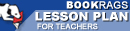Lesson Plans

# Journey Through Genius: The Great Theorems of Mathematics Test | Mid-Book Test - Easy

This set of Lesson Plans consists of approximately 142 pages of tests, essay questions, lessons, and other teaching materials.
 View a FREE sampleName: _________________________ Period: ___________________

This test consists of 15 multiple choice questions and 5 short answer questions.

## Multiple Choice Questions

1. Which of the following is true in modern math about twin primes?
(a) Their sum is always another prime number.
(b) We don't know if they are finite or infinite.
(c) They are not considered whole numbers.
(d) They are infinite.

2. What did Dunham consider extraordinary about the Elements?
(a) The content was not based on previous authors' work.
(b) How Hippocrates ordered the book.
(c) How geometric proofs were presented.
(d) The content was totally unique.

3. Which of the following becomes an important definition in mathematics that was first presented in Elements?
(a) 180 degree angle.
(b) Parallel line.
(c) Circle.
(d) Intersection.

4. Which of the following was NOT true about Archimedes, according to Dunham?
(a) He died as a soldier.
(b) Archimedes died because he refused to follow orders until he completed a math problem.
(c) His death was described as being by the hands of a Roman invader.
(d) He died during the fall of Syracuse.

5. What was the title of Cardano's book which contained the solution to the cubic?
(a) Tarsisia.
(b) Ars Magna.
(c) La Magnifica.
(d) Elements.

6. In general, what did Euclid's number theory describe?
(a) The nature of whole numbers.
(b) The nature of measuring geometry.
(c) The relationship of decimals to integers.
(d) The relationship of fractions to decimals.

7. What is true about prime numbers?
(a) Prime numbers can never be an odd number.
(b) That for every group of prime numbers, there exists at least one more prime.
(c) Prime numbers are not divisible by other numbers.
(d) Prime numbers can not exist in a finite series.

8. What do we know in modern times about Heron?
(a) We know he was an influencial scholar, but we don't know who his students were.
(b) We know he lived in Rome.
(c) We know he was a teacher and philosopher but much of his work has been lost.
(d) We know very little, but much of his work survives.

9. Which is one of the common notions presented in Elements?
(a) "Points with equal values can be connected with a line of equal value."
(b) "Things which are equal to the same thing are also equal to each other."
(c) "The inverse of a line makes a circle."
(d) "Things with are equal have an inverse that is equal."

10. What did Euclid do in his 48th proposition?
(a) Euclid proved the converse of the Pythagorean Theorem.
(b) Euclid demonstrated the faults of the Pythagorean Theorem.
(c) Euclid proved the Pythagorean Theorem.
(d) Euclid demonstrated how to use the Pythagorean Theorem.

11. Which of Euclid's postulates troubled many of the following generations of mathematicians?
(a) Euclid's postulate on creating an arc.
(b) Euclid's postulate on right triangles.
(c) Euclid's proof on right triangles.
(d) Euclid's postulate on parallel lines.

12. Where was Archimedes born?
(a) Olympia.
(b) Athens.
(c) Rome.
(d) Sicily.

13. Which of the following was true about Cardano, according to Dunham?
(a) He had three wives.
(b) He was a priest.
(c) He was not a mathematician.
(d) He was jailed for heresy.

14. Who acted as the gate keepers of knowledge?
(a) Greek philosophers.
(b) Roman emporers.
(c) Arabian scholars.
(d) Greek tradesman.

15. What was most useful about finding the square of a shape, before Hippocrates?
(a) It was useful in determining the distance between two points.
(b) It was useful in finding the area of circles.
(c) It was useful in finding the area of oddly shaped pieces of land.
(d) It was useful in creating simple elevation maps,

## Short Answer Questions

1. After working on pi, what did Archimedes continue with in his study of mathematics?

2. After Hippocrates, what shape did the Greeks attempt to square without success?

3. What was the bases of Hippocrates's proof ?

4. Who was Neil's Abel?

5. What did Euclid state about pi in Elements?

(see the answer keys)

 This section contains 723 words (approx. 3 pages at 300 words per page) View a FREE sampleCopyrightsJourney Through Genius: The Great Theorems of Mathematics from BookRags. (c)2019 BookRags, Inc. All rights reserved.
Follow Us on Facebook